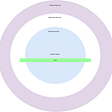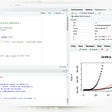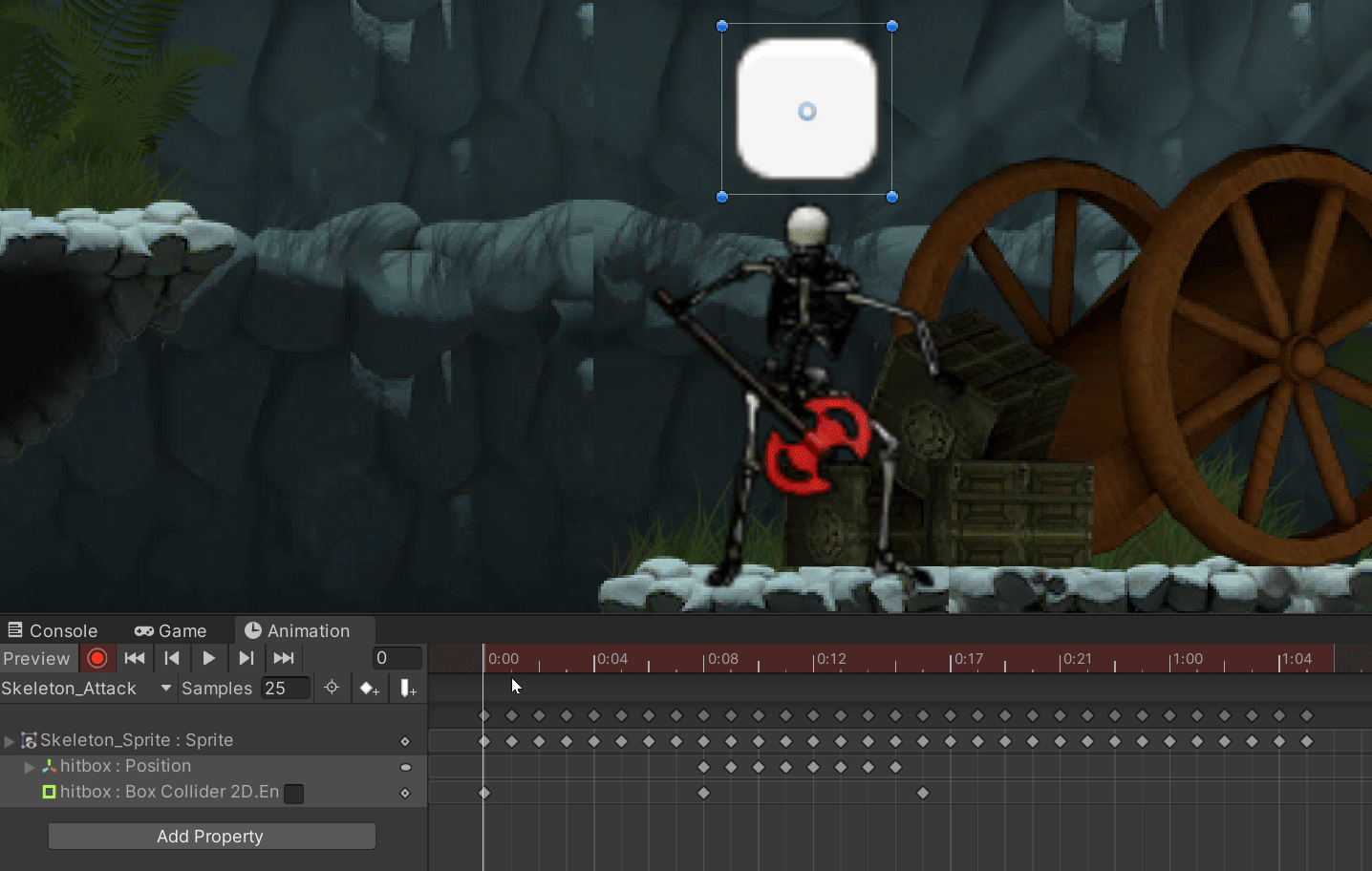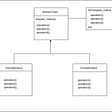# Integer to Roman — Day 93(Python)

Today’s question is a medium-tagged question in Leetcode. This question is asked in multiple coding interviews. Let us look into the problem statement.

12. Integer to Roman

Roman numerals are represented by seven different symbols: `I`, `V`, `X`, `L`, `C`, `D` and `M`.

`Symbol       ValueI             1V             5X             10L             50C             100D             500M             1000`

For example, `2` is written as `II` in Roman numeral, just two one's added together. `12` is written as `XII`, which is simply `X + II`. The number `27` is written as `XXVII`, which is `XX + V + II`.

Roman numerals are usually written largest to smallest from left to right. However, the numeral for four is not `IIII`. Instead, the number four is written as `IV`. Because the one is before the five we subtract it making four. The same principle applies to the number nine, which is written as `IX`. There are six instances where subtraction is used:

• `I` can be placed before `V` (5) and `X` (10) to make 4 and 9.
• `X` can be placed before `L` (50) and `C` (100) to make 40 and 90.
• `C` can be placed before `D` (500) and `M` (1000) to make 400 and 900.

Given an integer, convert it to a roman numeral.

Example 1:

`Input: num = 3Output: "III"`

Example 2:

`Input: num = 4Output: "IV"`

Example 3:

`Input: num = 9Output: "IX"`

Example 4:

`Input: num = 58Output: "LVIII"Explanation: L = 50, V = 5, III = 3.`

Example 5:

`Input: num = 1994Output: "MCMXCIV"Explanation: M = 1000, CM = 900, XC = 90 and IV = 4.`

Constraints:

• `1 <= num <= 3999`

The very first thing that we need to do is define a dictionary that stores the definition of different roman symbols. Apart from defined symbols, we will specific cases number also.

`d = {1000: 'M',      900: 'CM',      500: 'D',      400: 'CD',      100: 'C',       90: 'XC',      50: 'L',      40: 'XL',      10: 'X',        9: 'IX',        5: 'V',        4: 'IV',        1: 'I'}`

We need to make sure that the dictionary is defined in decreasing order.

Next, we will run a loop that starts from the first number in the dictionary. Check how many times the current input number is divisible by the dictionary key. This number will be the number of times the value of the respective dictionary key has to be repeated.

`For eg: If the input number is 2000. We start our loop from 1000 in the dictionary. 2000 is divisible by 1000 twice. The value of 1000 in the dictionary is "M". Hence "M" will be repeated twice.`

The roman numeral that we find in the above step will be stored in a result variable. Each time the loop is run, the input number is modulo by the current key in the dictionary.

Let us look into the code snippet.

`class Solution:    def intToRoman(self, num: int) -> str:        d = {1000: 'M',              900: 'CM',               500: 'D',               400: 'CD',               100: 'C',                90: 'XC',                50: 'L',                40: 'XL', 10: 'X', 9: 'IX', 5: 'V', 4: 'IV', 1: 'I'}                 res = ""                for i in d:            res += (num//i) * d[i]            num %= i        return res`

# Complexity analysis.

Time Complexity

The time complexity O(N) where is the number of keys in our dictionary. The number of keys in our dictionary depends on the range provided to us as a constraint for the input number.

Space Complexity

The space complexity is O(N) where N is the number of keys in our dictionary.

I had to look through the discussion board to understand how to solve this problem. The link to the discussion can be found here.

--

--

--

## More from Annamariya Tharayil

Software Engineer. Find me @ www.linkedin.com/in/annamariya-jt

Love podcasts or audiobooks? Learn on the go with our new app.

## Recommended from Medium## Python 3 — Mutable, Immutable… everything is object!## Software Architecture — The Onion Architecture## Sharing R code## Enemy Hitboxes## 13 Reasons Why Software Engineers Quit## Template design pattern and Strategy design pattern in Ruby.## Annamariya Tharayil

Software Engineer. Find me @ www.linkedin.com/in/annamariya-jt

## Replicated Acoustic Features Parkinson Database## Function in Python